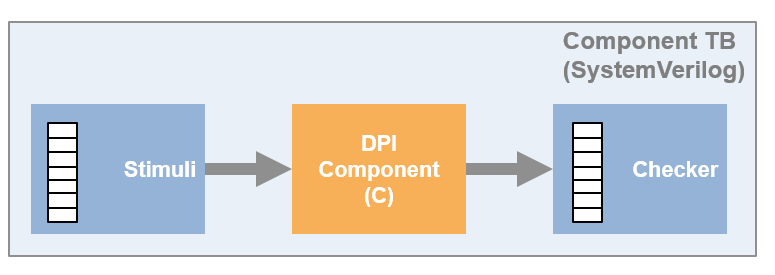## DPI Component Generation with MATLAB

You can export a MATLAB® function as a component with a direct programming interface (DPI) for use in a SystemVerilog simulation. Wrap generated C code with a DPI wrapper that communicates with a SystemVerilog thin interface function in a SystemVerilog simulation.

For MATLAB, you generate the component using the `dpigen` function.

Note

You must have a MATLAB Coder™ license to use this feature.

### Supported MATLAB Data Types

Supported MATLAB data types are converted to SystemVerilog data types, as shown in this table. When using the `dpigen` function, use the `PortsDataType` property to select ```Compatible C type```, `Logic vector`, or ```Bit vector``` data types.

Generated SystemVerilog Types

MATLABSystemVerilog
Compatible C TypeLogic VectorBit Vector
`uint8``byte unsigned``logic [7:0] ``bit [7:0] `
`uint16``shortint unsigned``logic [15:0] ``bit [15:0] `
`uint32``int unsigned``logic [31:0]``bit [31:0]`
`uint64``longint unsigned``logic [63:0]``bit [63:0]`
`int8``byte``logic signed [7:0]``bit signed [7:0]`
`int16``shortint``logic signed [15:0]``bit signed [15:0]`
`int32``int``logic signed [31:0]``bit signed [31:0]`
`int64``longint``logic signed [63:0]``bit signed [63:0]`
`logical``byte unsigned``logic [0:0]``bit [0:0]`
`fi` (fixed-point data type)

Depends on the fixed-point word length. If the fixed-point word length is greater than the host word size (for example, 64-bit vs. 32-bit), then this data type cannot be converted to a SystemVerilog data type by MATLAB Coder and you will get an error. If the fixed-point word length is less than or equal to the host word size, MATLAB Coder converts the fixed-point data type to a built-in C type.

`logic [n-1:0]`

```logic signed [n-1:0]```

The logic vector length (`n`) is equal to the `wordlength`. The sign is inherited from the fixed point type.

`bit [n-1:0]`

```bit signed [n-1:0]```

The bit vector length (`n`) is equal to the `wordlength`. The sign is inherited from the fixed point type.

`single``shortreal`
`double``real`
`complex`

The coder flattens complex signals into real and imaginary parts in the SystemVerilog component.

vectors, matrices

arrays

For example, a 4-by-2 matrix in MATLAB is converted into a one-dimensional array of eight elements in SystemVerilog. By default, the coder flattens matrices in column-major order. To change to row-major order, use the `-rowmajor` option with the `dpigen` function. For additional information, see Generate Code That Uses Row-Major Array Layout (MATLAB Coder).

`structure`

The coder flattens structure elements into separate ports in the SystemVerilog component.

enumerated data types`enum`

### Generated Shared Library

Function `dpigen` automatically compiles the shared library needed to run the exported DPI component in the SystemVerilog environment. The makefile that builds the shared library has the extension `_rtw.mk`. For example, for `fun.m`, the make file name is `fun_rtw.mk`.

During compilation, the function `dpigen` generates a library file.

• Windows® 64: `function_win64.dll`

• Linux®: `function.so`

`function` is the name of the MATLAB function you generated the DPI component from.

Note

If you use 64-bit MATLAB on Windows, you get a 64-bit DLL, which can be used only with a 64-bit HDL simulator.

### Generated Test Bench

Function `dpigen` also creates a test bench. You can use this test bench to verify that the generated SystemVerilog component is functionally equivalent to the original MATLAB function. The generator runs your MATLAB code to save input and output data vectors for use in the test bench. This test bench is not intended as a replacement for a system test bench for your own application. However, you can use the generated test bench as a starting example when creating your own system test bench.### Generated Outputs

• C and header files from your algorithm, generated by MATLAB Coder

• C and header files for the DPI wrapper, generated by HDL Verifier™

• SystemVerilog file that exposes the component and adds control signals

• SystemVerilog package file that contains all the function declarations of the DPI component

• SystemVerilog test bench (with the `-testbench` option)

• Data files used with the HDL simulator (with the `-testbench` option)

• HDL simulator scripts, such as `*.do` or `*.sh` (with the `-testbench` option)

• Makefile `*.mk`

### Generated SystemVerilog Wrapper

All SystemVerilog code generated by function `dpigen` contains a set of control signals and the `Initialize` function.

#### Generated Control Signals

• `clk`: synchronization clock

• `clk_enable`: clock enable

• `reset`: asynchronous reset

#### Generated Initialize Function

The `Initialize` function is called at the beginning of the simulation.

For example:

` import "DPI" function void DPI_Subsystem_initialize();`

If the asynchronous reset signal is high (goes from 0 to 1), `Initialize` is called again.

### Simulation Considerations

When simulating the DPI component in your HDL environment, `reset`, `clock`, and `clk_enable` behave as follows:

• When `clk_enable` is `0`, the DPI output function is not executed, and the output values are not updated.

• When `clk_enable` is `1` and `reset` is `0`, the DPI output function is executed on the positive edge of the clock signal.

• When `reset` is `1`, the internal state of the DPI component is set to its initial value. This action is equivalent to using the `clear` function in MATLAB to update persistent variables. Then output values then reflect the DPI component initial state and current input values. For more details on persistent variables, see Persistent Variables.

### Limitations

• Variable-sized arguments are not supported.

• Large fixed-point numbers that exceed the system word length are not supported.

• Some optimizations, such as constant folding, are not supported because they change the interface of the generated C function. For more information, see MATLAB Coder Optimizations in Generated Code (MATLAB Coder).

• HDL Verifier limits matrices and vectors to one-dimensional arrays in SystemVerilog. For example, a 4-by-2 matrix in MATLAB is converted to a one-dimensional array of eight elements in SystemVerilog.

• The PostCodegen callback in config objects is not supported.

## SupportGet trial now